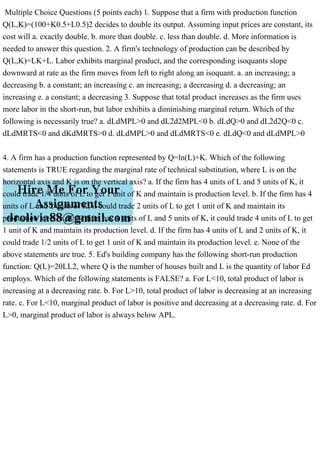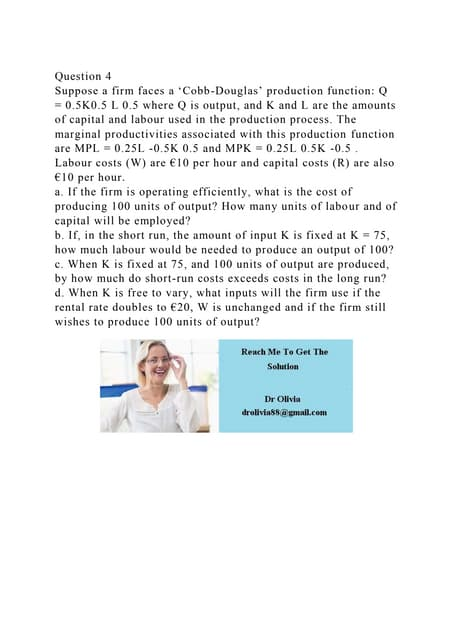Anúncio

# Multiple Choice Questions (5 points each) 1. Suppose that a firm with.pdf

1 de Apr de 2023Próximos SlideSharesQuestion 4Suppose a firm faces a ‘Cobb-Douglas’ production functio.docx
Carregando em ... 3
1 de 1
Anúncio

### Multiple Choice Questions (5 points each) 1. Suppose that a firm with.pdf

1. Multiple Choice Questions (5 points each) 1. Suppose that a firm with production function Q(L,K)=(100+K0.5+L0.5)2 decides to double its output. Assuming input prices are constant, its cost will a. exactly double. b. more than double. c. less than double. d. More information is needed to answer this question. 2. A firm's technology of production can be described by Q(L,K)=LK+L. Labor exhibits marginal product, and the corresponding isoquants slope downward at rate as the firm moves from left to right along an isoquant. a. an increasing; a decreasing b. a constant; an increasing c. an increasing; a decreasing d. a decreasing; an increasing e. a constant; a decreasing 3. Suppose that total product increases as the firm uses more labor in the short-run, but labor exhibits a diminishing marginal return. Which of the following is necessarily true? a. dLdMPL>0 and dL2d2MPL<0 b. dLdQ>0 and dL2d2Q<0 c. dLdMRTS<0 and dKdMRTS>0 d. dLdMPL>0 and dLdMRTS<0 e. dLdQ<0 and dLdMPL>0 4. A firm has a production function represented by Q=ln(L)+K. Which of the following statements is TRUE regarding the marginal rate of technical substitution, where L is on the horizontal axis and K is on the vertical axis? a. If the firm has 4 units of L and 5 units of K, it could trade 1/4 units of L to get 1 unit of K and maintain is production level. b. If the firm has 4 units of L and 2 units of K, it could trade 2 units of L to get 1 unit of K and maintain its production level. c. If the firm has 4 units of L and 5 units of K, it could trade 4 units of L to get 1 unit of K and maintain its production level. d. If the firm has 4 units of L and 2 units of K, it could trade 1/2 units of L to get 1 unit of K and maintain its production level. e. None of the above statements are true. 5. Ed's building company has the following short-run production function: Q(L)=20LL2, where Q is the number of houses built and L is the quantity of labor Ed employs. Which of the following statements is FALSE? a. For L<10, total product of labor is increasing at a decreasing rate. b. For L>10, total product of labor is decreasing at an increasing rate. c. For L<10, marginal product of labor is positive and decreasing at a decreasing rate. d. For L>0, marginal product of labor is always below APL.
Anúncio